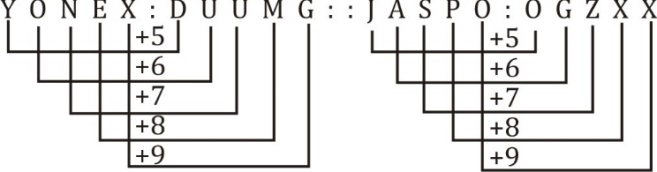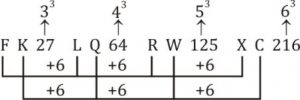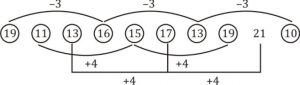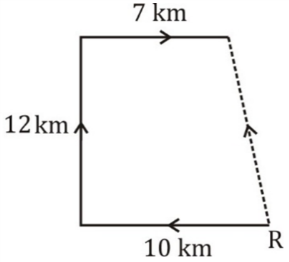# Reasoning Quiz [beginner level] For SSC CGL : 28th December

Q1. Mathematics : Formulas : : Chemistry : ?
(a) Reactions
(b) Organisms
(c) Theorems
(d) Gravity

Q2. YONEX : DUUMG : : JASPO : ?
(a) OGZXX
(b) OXXZF
(c) OZTYY
(d) OZXXG

Q3. 49 : 169 : : 66 : ?
(a) 126
(b) 132
(c) 144
(d) 162

Direction(4-6): In the following question, select the odd word from the given alternatives.
Q4. (a) Mobile
(b) Computer
(c) Fountain Pen
(d) Television

Q5. (a) BN – P
(b) GI – R
(c) LM – Y
(d) TA – U

Q6. (a) (143, 64)
(b) (232, 49)
(c) (719, 289)
(d) (462, 169)

Q7. Arrange the given words in the sequence in which they occur in the dictionary.
1. Exacts 2. Exotic 3. Exactly
4. Exacerbate 5. Exhaust
(a) 43152
(b) 43251
(c) 53421
(d) 54312

Q8. A series is given with one term missing. Select the correct alternative from the given ones that will complete the series.
FK27, LQ64, RW125, ?
(a) CX216
(b) XB216
(c) XC216
(d) YB343

Q9. In the following question, select the missing number from the given series.
19, 11, 13, 16, 15, 17, 13, 19, 21, ?
(a) 10
(b) 11
(c) 12
(d) 15

Q10. Punit starting from point R walked straight 10 km west, then turned right and walked 12 km and again turned right and walked straight 7 km. In which direction is he from point R?
(a) North-East
(b) North-West
(c) South-East
(d) South-West

Solutions:

S1. Ans. (a);
Sol. Mathematics contain formulas
Chemistry contain reactions

S2. Ans. (a);
Sol.S3. Ans. (c);
Sol. 49 = 4 + 9 = 13² = 169
66 = 6 + 6 = 12² = 144

S4. Ans. (c);
Sol. Except fountain pen, rest three are electronics items.

S5. Ans. (b);
Sol. B N = 2 + 14 = 16 = P
L M = 12 + 13 = 25 = Y
T A = 20 + 1 = 21 = U
G I = 7 + 9 = 16 = P ≠ R

S6.Ans. (d);
Sol. 143 = 1 + 4 + 3 = 8² = 64
232 = 2 + 3 + 2 = 7² = 49
719 = 7 + 1 + 9 = 17² = 289
462 = 4 + 6 + 2 = 12² = 144 ≠ 169

S7. Ans. (a);
Sol. 4. Exacerbate
3. Exactly
1. Exacts
5. Exhaust
2. Exotic

S8. Ans. (c);
Sol.S9. Ans. (a);
Sol.S10. Ans. (b);
Sol.He is in North-west direction from point R.

### Are You Preparing For SSC Exams? Register Here to get Free Study Material

Click here for best SSC CGL mock tests, video course, live batches, books or eBooks

×

Thank You, Your details have been submitted we will get back to you.Join India largest learning distination

What You Will get ?

•Job Alerts
•Daily Quizes
•Subject-Wise Quizes
•Current Affairs
•previous year question papers
•Doubt Solving session

ORJoin India largest learning distination

What You Will get ?

•Job Alerts
•Daily Quizes
•Subject-Wise Quizes
•Current Affairs
•previous year question papers
•Doubt Solving session

ORJoin India largest learning distination

What You Will get ?

•Job Alerts
•Daily Quizes
•Subject-Wise Quizes
•Current Affairs
•previous year question papers
•Doubt Solving session

Enter the email address associated with your account, and we'll email you an OTP to verify it's you.Join India largest learning distination

What You Will get ?

•Job Alerts
•Daily Quizes
•Subject-Wise Quizes
•Current Affairs
•previous year question papers
•Doubt Solving session

## Enter OTP

Please enter the OTP sent to
/6

Did not recive OTP?

Resend in 60sJoin India largest learning distination

What You Will get ?

•Job Alerts
•Daily Quizes
•Subject-Wise Quizes
•Current Affairs
•previous year question papers
•Doubt Solving sessionJoin India largest learning distination

What You Will get ?

•Job Alerts
•Daily Quizes
•Subject-Wise Quizes
•Current Affairs
•previous year question papers
•Doubt Solving session

## Almost there

+91Join India largest learning distination

What You Will get ?

•Job Alerts
•Daily Quizes
•Subject-Wise Quizes
•Current Affairs
•previous year question papers
•Doubt Solving session

## Enter OTP

Please enter the OTP sent to Edit Number

Did not recive OTP?

Resend 60

## By skipping this step you will not recieve any free content avalaible on adda247, also you will miss onto notification and job alerts

Are you sure you want to skip this step?

## By skipping this step you will not recieve any free content avalaible on adda247, also you will miss onto notification and job alerts

Are you sure you want to skip this step?